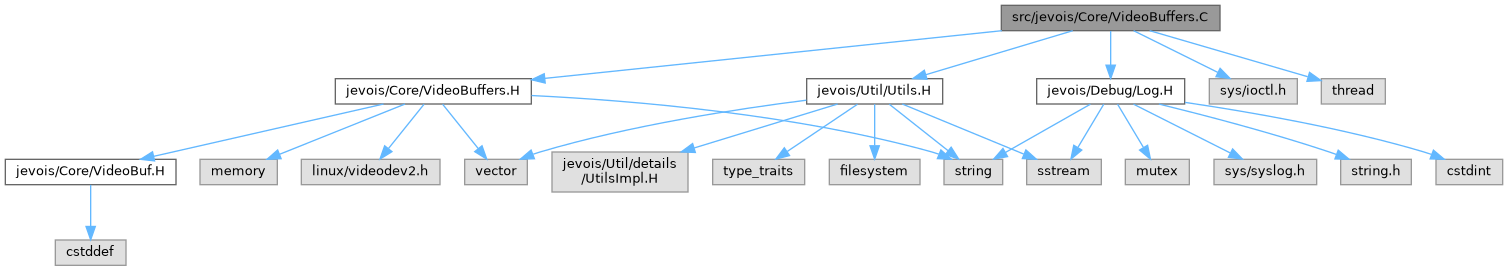JeVois  1.19
JeVois Smart Embedded Machine Vision Toolkit
 Share this page: TweetVideoBuffers.C File Reference
`#include <jevois/Core/VideoBuffers.H>`
`#include <jevois/Util/Utils.H>`
`#include <jevois/Debug/Log.H>`
`#include <sys/ioctl.h>`
`#include <thread>`
Include dependency graph for VideoBuffers.C:Go to the source code of this file.

## Macros

#define FDLDEBUG(msg)   LDEBUG('[' << itsFd << ':' << itsName << "] " << msg)

#define FDLINFO(msg)   LINFO('[' << itsFd << ':' << itsName << "] " << msg)

#define FDLERROR(msg)   LERROR('[' << itsFd << ':' << itsName << "] " << msg)

#define FDLFATAL(msg)   LFATAL('[' << itsFd << ':' << itsName << "] " << msg)

## ◆ FDLDEBUG

 #define FDLDEBUG ( msg ) LDEBUG('[' << itsFd << ':' << itsName << "] " << msg)

Definition at line 24 of file VideoBuffers.C.

## ◆ FDLERROR

 #define FDLERROR ( msg ) LERROR('[' << itsFd << ':' << itsName << "] " << msg)

Definition at line 26 of file VideoBuffers.C.

## ◆ FDLFATAL

 #define FDLFATAL ( msg ) LFATAL('[' << itsFd << ':' << itsName << "] " << msg)

Definition at line 27 of file VideoBuffers.C.

## ◆ FDLINFO

 #define FDLINFO ( msg ) LINFO('[' << itsFd << ':' << itsName << "] " << msg)

Definition at line 25 of file VideoBuffers.C.